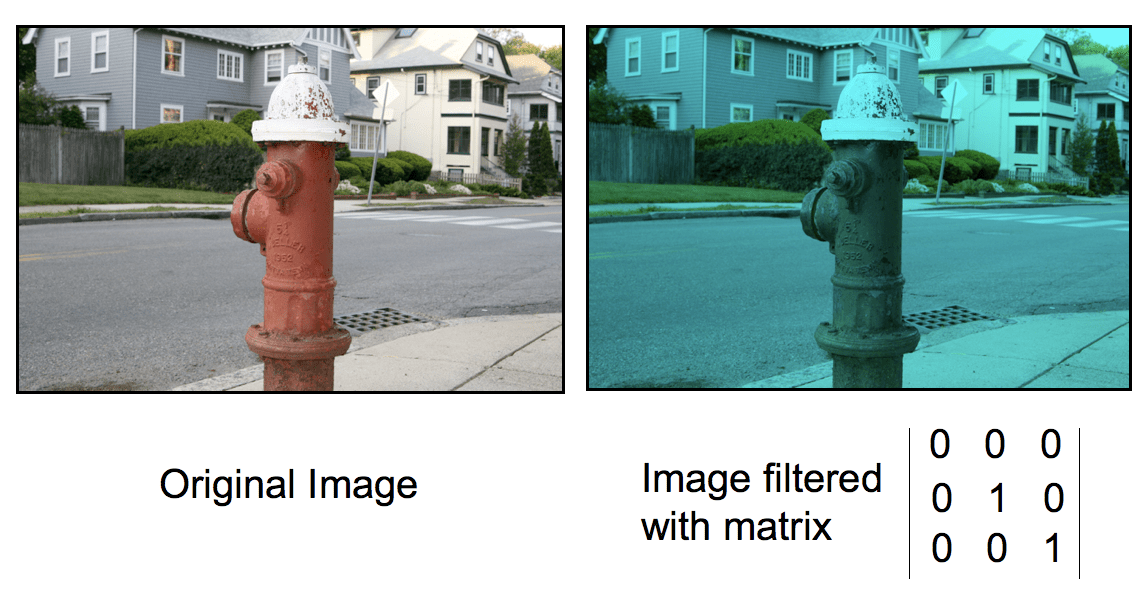Color filters allow you to alter an image by … well … filtering out colors from an image. While Processing has some filters built-in, it’s often handy to be able to create your own custom filters. For example, the site Anaglyph Methods Comparison details a number of different filters that are handy in creating 3D images.

As you’ll see from the site, filters are basically mathematical transformations that are applied to the red, green, and blue components of the pixels in an image. To create a filter like the ones on the stereo anaglyph site, we need to pull each pixel in the original image, apply a matrix operation to its color components, and then use this new value in the corresponding pixel in the new image. This Codebox shows you how to create your own filters like these.

First, though, a bit of review for the (potentially) hairy looking math. A matrix is a 2 dimensional array of numbers called elements. Matrices are used in many different applications, representing sets of equations, transformations to 3D objects, and color filters (as in this example).

A matrix is usually characterized by its dimensions: the number of elements in its rows (the numbers going across ) and columns (the numbers going up and down). By convention, the number of rows always comes first. The following figure shows 2 matrices. On the left is a 2 row by 3 matrix, which is generally just called a 2×3 matrix. On the right is a 3 row by 2 column matrix, also called a 3×2 matrix:To multiply two matrices together (let’s call them matrix A and matrix B), the number of columns in matrix A must equal the number of rows in matrix B. This is critical — if this isn’t true, then the multiplication is not defined. (This is sort of like dividing by zero — it’s simply impossible.)

Assuming this criteria is met, the product of the two matrices (lets call it matrix C) will have the same number of rows as matrix A and the same number of columns as matrix B. The elements in matrix C are equal to the sum of the products of the corresponding column element in A times the row elements in B. This is a bit tricky, but the following figure should (hopefully!) make this a bit clearer:The following sketch, matrix_mult.pde, illustrates how to do the operation in Processing:

When you run the sketch, you should see the following output:

 = 2.0
 = 0.0
 = 5.5
 = 2.0

So, with the basic math out of the way, we’re ready to write the filter code. The remaining wrinkle is that images in Processing are held in one dimensional arrays of colors, rather than 2 dimensional arrays of (x,y) coordinates, as explained in this great Images and Pixels tutorial by Daniel Shiffman. In the tutorial, he gives a nice formula that you can use to map an (x,y) coordinate to the position in the image array:

position in array = x + IMAGE_WIDTH * y

The following figure should help illustrate most of the concepts at work here:Without further ado, here’s a sketch called filter.pde to implement the filter through matrix-multiplication:

More:
Check out all of the Codebox columns here

In the Maker Shed:Getting Started with Processing
Learn computer programming the easy way with Processing, a simple language that lets you use code to create drawings, animation, and interactive graphics. Programming courses usually start with theory,but this book lets you jump right into creative and fun projects. It’s ideal for anyone who wants to learn basic programming, and serves as a simple introduction to graphics for people with some programming skills.

Tagged